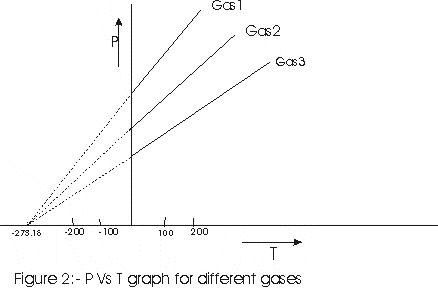# Ideal Gas Equation And Absolute Temperature

## Ideal Gas Equation

Pressure of all the gases changes with the temperature in a similar fashion for low temperature. Also many properties of gases are common at low pressure. The pressure, volume and temperature in kelvin of such gases obey the equation \begin{equation} PV=nRT \tag{1} \end{equation} where $n$ is amount of gas in number of moles and is given as , $$n = \left( {\frac{{{\textrm{Total number of molecules in given mass of gas}}}}{{{\textrm{Avagadro Number}}{{\textrm({N}}_A})}}} \right)$$ where, $N_{A}=6.023\times 10_{23}$ $R$ is universal gas constant and its value is $R=8.316$ J/mole-K. Equation (1) is known ideal gas equation and a gas obeying this equation is known ideal gas equation.

## Absolute Temperature

Suppose we calibrate two thermometers, for example, the liquid in a tube and a resistance thermometer. Here they agree at $0^0C$ and $100^0C$, but they may not agree exactly at intermediate temperatures. Any temperature scale defined in this way depends on the specific properties of the material used. We want to define a temperature scale which does not depend on the properties of a particular material. Here we will discuss a thermometer that comes close to ideal. That thermometer is a Gas thermometer. A thermometer that uses a gas gives the same reading regardless of the gas used.
Principle of a gas thermometer :- The pressure of a gas at constant volume increases with temperature.
In gas thermometer , a quantity of gas is placed in a constant volume container. Then we measure its pressure. To calibrate a constant volume gas thermometer, we measure pressure at $0^C$ and $100^C$. We now plot these points on a graph and draw straight line between these two points. From this graph we can read the temperature corresponding to other pressure. Figure below show the result of three such experiments with different gases.It is found that when graph were extrapolated for temperature below $0^{\circ}C$, pressure comes out to be zero at $-273^{\circ}C$ and this is the lowest temperature. Lord kelvin suggested that instead of $0^{\circ}C$ which is the melting point of ice,$-273^{\circ}C$ should be regarded as the zero of the temperature scale. Such a scale of temperature is absolute scale of temperature and $-273.15^{\circ} C$ as absolute zero of this new scale and is denoted as $0K$ and steam point in this scale correspond to $373.15 K$. From various experiments it turns out that this temperature is same for many different gases, at least in the limit of very low gas density. In gas thermometer different gases are used for measuring high temperatures and temperature reading are found to be independent of the nature of the gas used.
Gas thermometer can also define Celsius scale. If $P_{0}$ is pressure of gas at ice point and $P_{100}$ is pressure of gas at steam point then temperature T corresponding to a pressure P of gas is defined by, $$T=\frac{(P-P_{0})100}{(P_{100}-P_{0})}$$